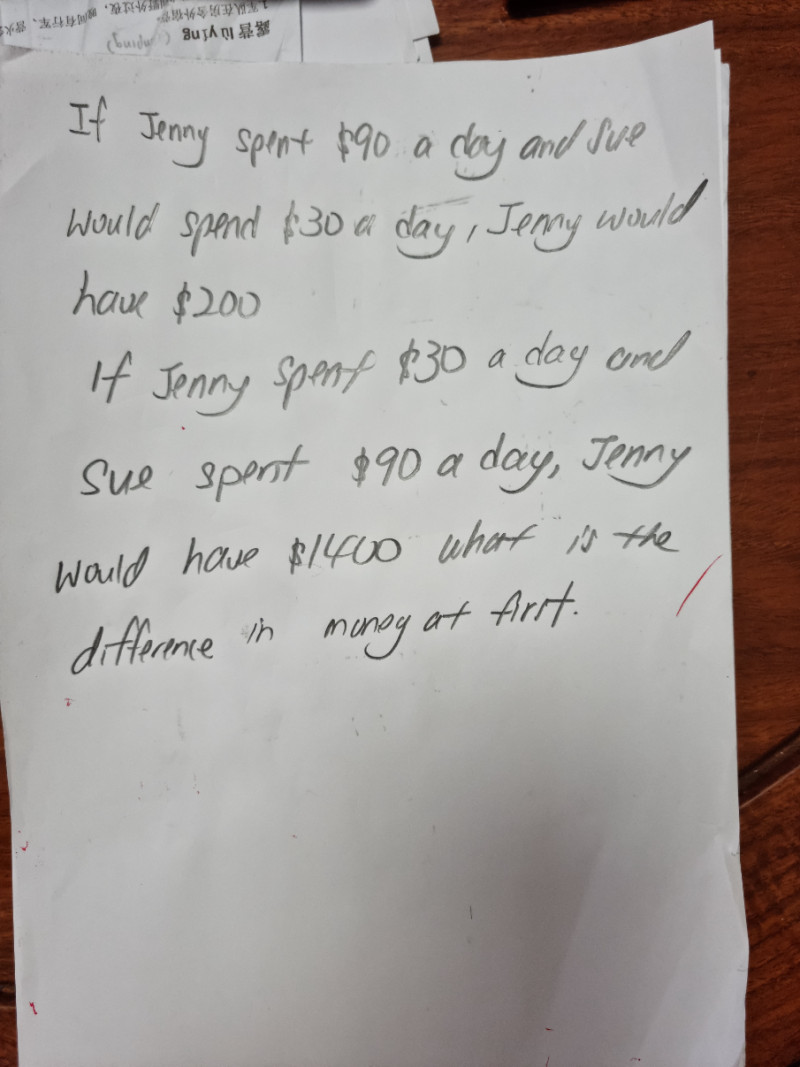# QuestionThank you

Scenario 1:  J left \$200

J              S

90     :    30

3        :      1

9u        :      3u    <—–make S the same ratio because it is implied S has no money left in both cases.

Scenario 1:  J left \$1400

J              S

30     :    90

u        :      3u

Then 9u-u = 1400-200

8u = 1200

u = 150

So at first S = 3u = 3 x 150 = 450 and J = 1400+ 150 = 1550  or  J = 9×150 + 200 = 1550

Hence difference in money initially = 1550-450 = \$1100

0 Replies 2 Likes ✔Accepted Answer